# Introduction to Python Module Class 11 Computer Science

### What is module in Python?

Modules are simply files with the “. py” extension containing Python code that can be imported inside another Python Program. A module can contain executable statements as well as function definitions. A module allows you to logically organise your python code.

### User-Defined Modules in Python

You can create your own functions and classes, put them inside modules and voila! You can now include hundreds of lines of code into any program just by writing a simple import statement. To create a module, just put the code inside a . py file.

### Creating a Module in Python:

In Python , the modules or User-Defined functions are created with def keyword. The general syntax is:

def module_name/function_name():

python program statements

# Program to create a module in Python.

```def sum():
a=int(input("Enter first number"))
b=int(input("Enter second number"))
result=a+b
print("sum of %d and %d is %d"%(a,b,result))
```

Now I am saving the above python code as sum_module.py.

### Importing Python Module in Same Program:

Now we will import the above created module.

```>>> import sum_module
>>> sum_module.sum()
Enter first number45
Enter second number34
sum of 45 and 34 is 79
>>>```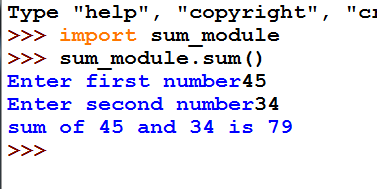### Importing Python Module in another Program

Let we create a simple calculator module/function with three basic functions add, multiplication and division (+,* ,/). We will save the python file as calculator.py.

```# creating module/function with three airthmetic operations (+,* and /)
def sum(a,b):
result= a+b
return result
def mul(a,b):
result= a*b
return result
def div(a,b):
result= a/b
return result
```

Now we will create an another python program named mathclc.py , from where we access the functions of above program calculator.py.

```# importing calculator module
import calculator
a=int(input("Enter first number"))
b=int(input("Enter second number"))
print ("sum of entered numbers",calculator.sum(a,b))
print ("multiplication of entered numbers",calculator.mul(a,b))
print ("division of entered numbers",calculator.div(a,b))
```

Output:

```Enter first number10
Enter second number2
('sum of entered numbers', 12)
('multiplication of entered numbers', 20)
('division of entered numbers', 5)
>>>```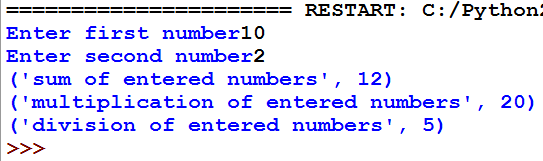## The from import Statement

With this method we can import some specific function definitions from a module without importing the whole module.

Syntax:

from module_name import function_name

For better understanding we will implement this method in above created module calculator.py.

```>>> from calculator import sum
>>> sum(10,12)
22
>>>```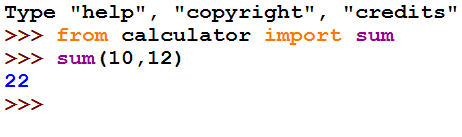### The from module import * Statement

In this method we import all the functions from a module into the current program. In this method (*) means to import all objects into the calling program.

Syntax:

from module_name import *

In previous programs we created calculator.py module. Now we will create another module area.py to calculate area of rectangle and will import these two modules in another program named test.py.

# Python function/ module to calculate area of rectangle area.py

```# module/function to calculate area of rectangle
def react_area(l,w):
result=(l*w)
return result

```

# Python module test.py in which we import above modules calculator.py andarea.py.

```from calculator import *
from area import *
print("area of rectangle",react_area(5,3))
print("sum of entered numbers is",sum(5,3))
```

```Output:

('area of rectangle', 15)
('sum of entered numbers is', 8)
>>>```

### Built in Modules/ Standard Library in Python

A library is just a module that contains some useful definitions. Python has a large variety of standard library modules.

Some of them are math module, random module, datetime module and statistics module etc.

In this section we will only cover math module.

## math Module:

Python has a math module that provides most of the mathematical and trigonometric functions. To use math module you just have to put a line import math at the top of your python code.

How to use:

Syntax:

import math

print math.function_name(variable)

math module has the following functions.

 Function Description pi Mathematical constant, the ratio of circumference of a circle to it’s diameter (3.14159…) e mathematical constant e (2.71828…) sqrt(x) Returns the square root of x ceil(x) Returns the smallest integer greater than or equal to x floor(x) Returns the largest integer less than or equal to x pow(x,y) Returns x raised to the power y fabs(x) Returns the absolute value of x sin(x) Returns the sine of x cos(x) Returns the cosine of x tan(x) Returns the tangent of x

## Implementation of math module

```import math
print(math.pi)
print(math.e)
print(math.sqrt(25))
print(math.ceil(5))
print(math.floor(8))
print(math.pow(4,3))
print(math.fabs(3))
print(math.sin(30))
print(math.cos(60))
print(math.tan(45))
```

Output:

```3.14159265359
2.71828182846
5.0
5.0
8.0
64.0
3.0
-0.988031624093
-0.952412980415
1.61977519054
>>>```

Screenshots: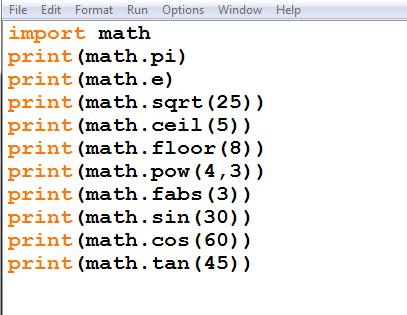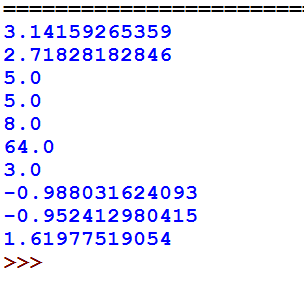## Check Here: Random Function in Python Class 11 Notes#### By cbsepython

A complete solution for the students of class 9 to 12 having subject Information Technology (402), Computer Science (083). Explore our website for all the useful content as Topic wise notes, Solved QNA, MCQs, Projects and Quiz related to the latest syllabus.